﻿ 起动负浮力射流中涡环形成与演化的实验研究 On the Vortex Ring Formation and Evolution in Negatively Buoyant Jet

Applied Physics
Vol. 09  No. 04 ( 2019 ), Article ID: 29853 , 15 pages
10.12677/APP.2019.94023

On the Vortex Ring Formation and Evolution in Negatively Buoyant Jet

Shengwen Chen, Lei Gao*

School of Aeronautics and Astronautics, Sichuan University, Chengdu Sichuan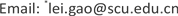Received: Apr. 2nd, 2019; accepted: Apr. 17th, 2019; published: Apr. 24th, 2019ABSTRACT

In this paper, the formation and evolution of negative buoyancy vortex rings are studied experimentally. For small stroke ratio (L/D = 2, 3) cases, the evolution of the buoyant vortex ring in the near field is investigated. It is found that as Richardson number Ri increases, the growth rates of the vortex ring radius, as well as its translational velocity are reduced. The kinetic parameters of the buoyant vortex ring, i.e., the hydrodynamic impulse and circulation, are also diminished by Ri. The impulse and velocity of the buoyancy vortex ring are obtained and compared with those of the thin core vortex ring model. It is found that the buoyant vortex ring conforms to the characteristics of thin core vortex ring model.

Keywords:Negatively Buoyant Jet, Vortex Ring, Richardson NumberCopyright © 2019 by author(s) and Hans Publishers Inc.1. 引言

Maxworth   通过活塞装置产生涡环并研究了其生成与演化的过程。Didden  建立了Slug模型描述了射流产生的涡环的物理特征。Shariff等  和Lim等  对之前的在静止、非分层环境中涡环的动力学特征进行了总结。Gharib等  通过对活塞–圆管装置产生的轴对称涡环的形成过程进行研究，发现了涡环

$Fr=R{i}^{–1}Ri=D\frac{\Delta \rho }{\rho }g/{U}_{p}^{2}$ (1)

$Fr>1$ 时，随着Fr的增加，形成数也随着增加，最后达到均质射流的值3~4左右。也就是说相对于均质射流，负浮力射流的形成数减少了。与之相对应的Wang  等通过对正浮力射流的数值模拟研究，发现正的浮力增加了其形成数。Gao  等通过数值模拟，研究了 $-0.06 的浮力射流，并确定了形成数F与分离数S随Ri变化的规律。其中，分离数S是当尾流与前导涡环完全分离时的无量纲时间。在理论研究上，Shusser与Gharib  对起动浮力羽流中涡环演化建立了积分模型，对其浮力涡环的形成数进行了预测。

2. 实验部分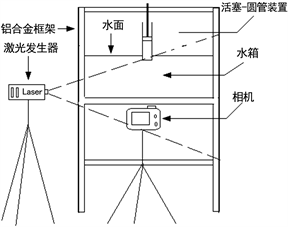Figure 1. Starting buoyancy jet device

3. 结果与分析

3.1. 涡量场与速度场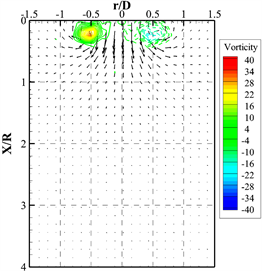(a) t* = 1.05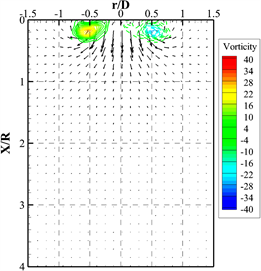(d) t* = 1.05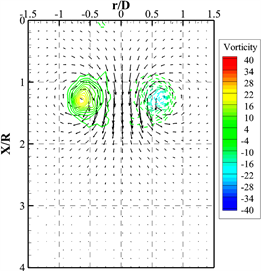(b) t* = 4.05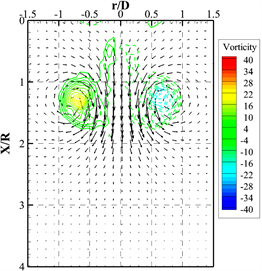(e) t* = 4.05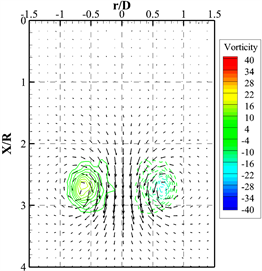(c) t* = 7.8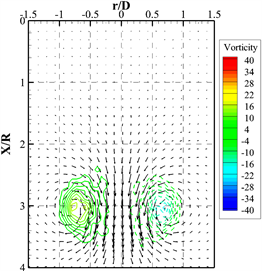(f) t* = 7.8

Figure 2. (a) - (e) Vorticity contours and velocity vectors at different moments, while Ri = 0, L/D = 2; (f) - (j) Vorticity contours and velocity vectors at different moments, while Ri = 0, L/D = 3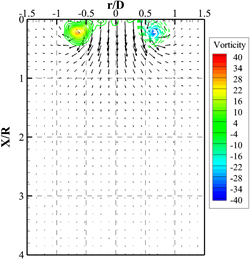(a) t* = 1.05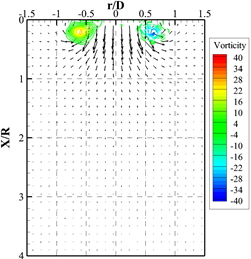(d) t* = 1.05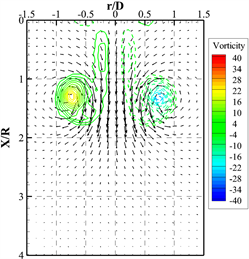(b) t* = 4.05(e) t* = 4.05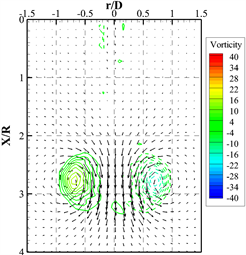(c) t* = 7.8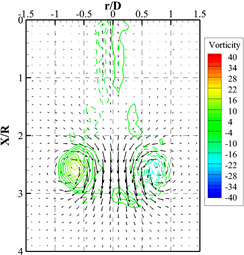(f) t* = 7.8

Figure 3. (a) - (c) Vorticity contours and velocity vectors at different moments, while Ri = −0.05, L/D = 3; (d) - (f) Vorticity contours and velocity vectors at different moments, while Ri = −0.1, L/D = 3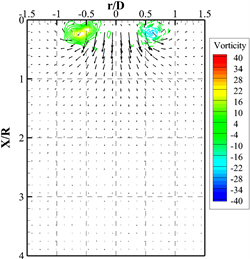(a) t* = 1.05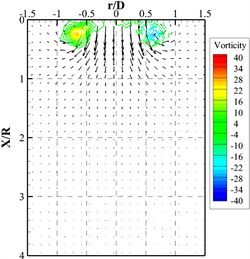(d) t* = 1.05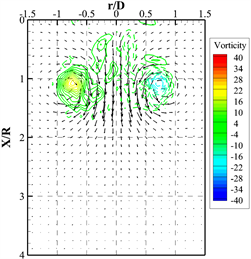(b) t* = 4.05(e) t* = 4.05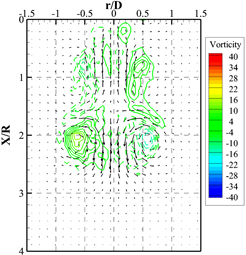(c) t* = 7.8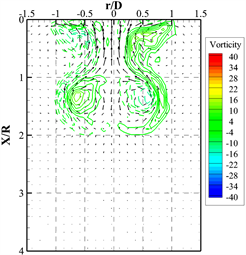(f) t* = 7.8

Figure 4. (a) - (c) Vorticity contours and velocity vectors at different moments, while Ri = −0.2, L/D = 3; (d) - (f) Vorticity contours and velocity vectors at different moments, while Ri = −0.3, L/D = 3(a) t* = 3.3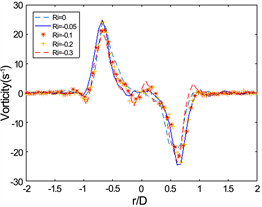(b) t* = 4.05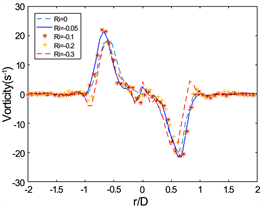(c) t* = 4.3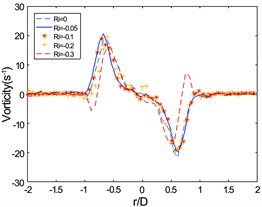(d) t* = 5.55

Figure 5. The vorticity distribution at different moments through the X/R position of the vortex core, while L/D = 2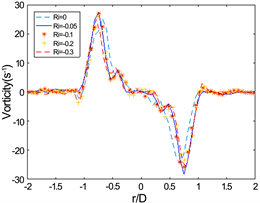(a) t* = 3.3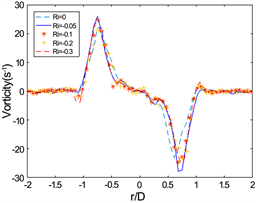(b) t* = 4.05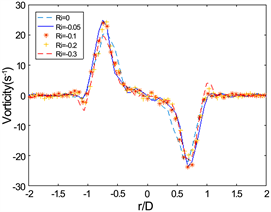(c) t* = 4.3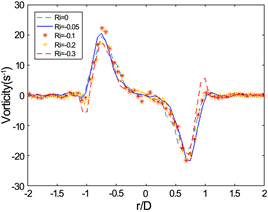(d) t* = 5.55

Figure 6. The vorticity distribution at different moments through the X/R position of the vortex core, while L/D = 3

3.2. 涡核位置与涡环半径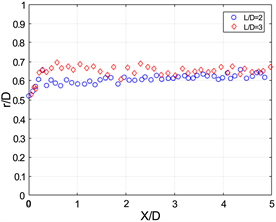(a) Ri = 0(b) Ri = −0.05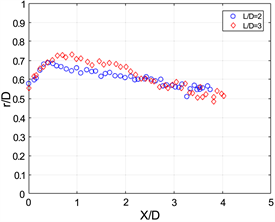(c) Ri = −0.1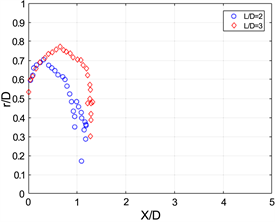(d) Ri = −0.3

Figure 7. Vortex core position at different Ri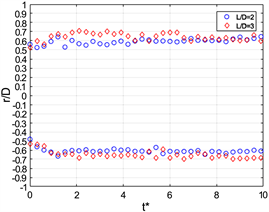(a) Ri = 0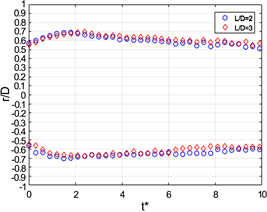(b) Ri = −0.05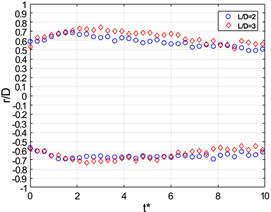(c) Ri = −0.1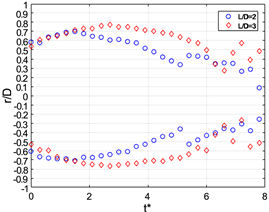(d) Ri = −0.3

Figure 8. Dimensional vortex ring radius versus formation time t*

3.3. 涡环的环量、冲量与速度

$I=\text{π}{\int }^{\text{​}}\omega {r}^{2}\text{d}x\text{d}r$ (2)

$\Gamma ={\int }^{\text{​}}\omega {r}^{2}\text{d}x\text{d}r$ (3)

${\Gamma }^{\prime }={U}_{p}^{2}t/2$ (4)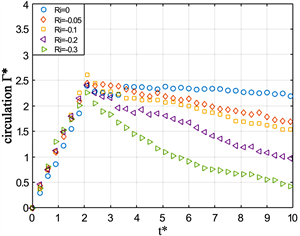(a) L/D = 2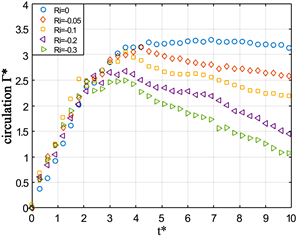(b) L/D = 3

Figure 9. Dimensional circulation Г* versus formation time t* at different stroke ratio

$I=\rho \text{π}{R}^{2}$ (5)

$V=\frac{\Gamma }{4\text{π}R}\left(\mathrm{ln}\frac{8}{\epsilon }-\frac{1}{4}\right)$ (6)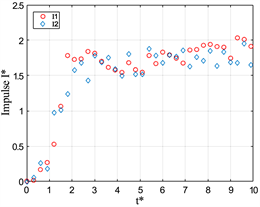(a) Ri = 0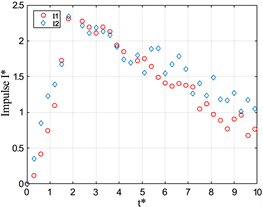(b) Ri = −0.05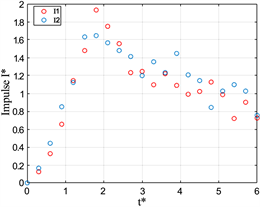(c) Ri = −0.1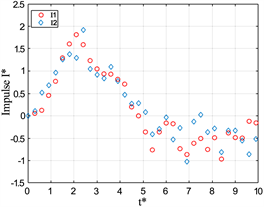(d) Ri = −0.3

Figure 10. Dimensional impulse I* versus formation time t* at L/D = 2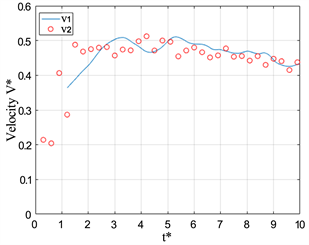(a) Ri = 0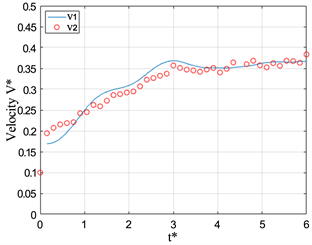(b) Ri = −0.05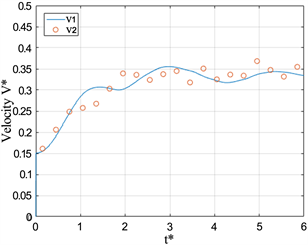(c) Ri = −0.1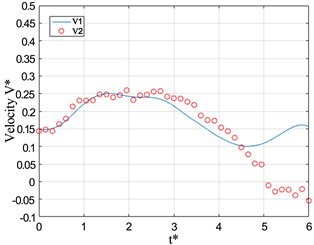(d) Ri = −0.3

Figure 11. Dimensional Velocity V* versus formation time t* at L/D = 2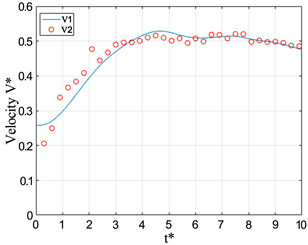(a) Ri = 0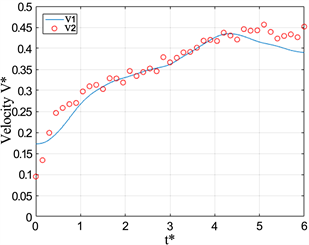(b) Ri = −0.05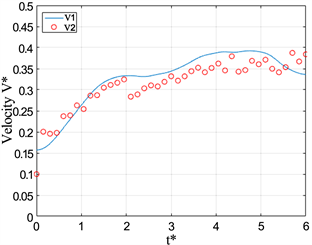(c) Ri = −0.1(d) Ri = −0.3

Figure 12. Dimensional Velocity V* versus formation time t* at L/D = 3

4. 结语

On the Vortex Ring Formation and Evolution in Negatively Buoyant Jet[J]. 应用物理, 2019, 09(04): 182-196. https://doi.org/10.12677/APP.2019.94023

1. 1. 向阳. 涡环演化及其物理特征的研究[D]: [硕士学位论文]. 上海: 上海交通大学, 2013.

2. 2. Maxworthy, T. (1972) The Structure and Stability of Vortex Rings. Journal of Fluid Mechanics, 51, 15-32. https://doi.org/10.1017/S0022112072001041

3. 3. Maxworthy, T. (1977) Some Experimental Studies of Vortex Rings. Journal of Fluid Mechanics Digital Archive, 81, 31. https://doi.org/10.1017/S0022112077002171

4. 4. Didden, N. (1979) On the Formation of Vortex Rings: Roll-ing-Up and Production of Circulation. Zeitschrift fur Angewandte Mathematik und Physik (ZAMP), 30, 101-116. https://doi.org/10.1007/BF01597484

5. 5. Shariff, K. and Leonard, A. (1992) Vortex Rings. Annual Review of Fluid Mechanics, 24, 235-279. https://doi.org/10.1146/annurev.fl.24.010192.001315

6. 6. Lim, T.T. and Nickels, T.B. (1995) Vortex Rings. In: Green, S.I., Ed., Fluid Vortices, Kluwer Academic Publisher, Dordrecht, 95-153. https://doi.org/10.1007/978-94-011-0249-0_4

7. 7. Gharib, M., Rambod, E. and Shariff, K. (1998) A Universal Time Scale for Vortex Ring Formation. Journal of Fluid Mechanics, 360, 121-140. https://doi.org/10.1017/S0022112097008410

8. 8. Krueger, P.S. and Gharib, M. (2003) The Significance of Vortex Ring Formation to the Impulse and Thrust of a Starting Jet. Physics of Fluids, 15, 1271. https://doi.org/10.1063/1.1564600

9. 9. Weigand, A. and Gharib, M. (1997) On the Evolution of Laminar Vortex Rings. Experiments in Fluids, 22, 447-457. https://doi.org/10.1007/s003480050071

10. 10. Norbury, J. (1973) A Family of Steady Vortex Rings. Journal of Fluid Mechanics, 57, 417-431. https://doi.org/10.1017/S0022112073001266

11. 11. Fraenkel, L.E. (1970) On Steady Vortex Rings of Small Cross-Section in an Ideal Fluid. Proceedings of the Royal Society of London. Series A, 316, 29-62. https://doi.org/10.1098/rspa.1970.0065

12. 12. Saffman, P.G. (1978) The Number of Waves on Unstable Vortex Rings. Journal of Fluid Mechanics, 84, 625-639. https://doi.org/10.1017/S0022112078000385

13. 13. Sullivan, I.S., Niemela, J.J., Hershberger, R.E., Bolster, D. and Donnelly, R.J. (2008) Dynamics of Thin Vortex Rings. Journal of Fluid Mechanics, 609, 319-347. https://doi.org/10.1017/S0022112008002292

14. 14. Turner, J.S. (1957) Buoyant Vortex Rings. Proceedings A, 239, 61-75. https://doi.org/10.1098/rspa.1957.0022

15. 15. Turner, J.S. (1962) The “Starting Plume” in Neutral Sur-roundings. Journal of Fluid Mechanics Digital Archive, 13, 13. https://doi.org/10.1017/S0022112062000762

16. 16. 郑毅, 应纯同, 李磊. 稳定大气条件下爆炸烟云的数值模拟研究[J]. 力学与实践, 2009, 31(3): 31-34. https://doi.org/10.1097/01.COT.0000360409.30578.0d

17. 17. Pottebaum, T.S. and Gharib, M. (2004) The Pinch-Off Process in a Starting Buoyant Plume. Experiments in Fluids, 3, 87-94. https://doi.org/10.1007/s00348-004-0788-0

18. 18. Bond, D. and Johari, H. (2005) Effects of Initial Geometry on the Development of Thermals. Experiments in Fluids, 39, 591-601. https://doi.org/10.1007/s00348-005-0997-1

19. 19. Marugan-Cruz, C., Rodriguez-Rodriguez, J. and Martinez-Bazan, C. (2009) Negatively Buoyant Starting Jets. Physics of Fluids, 21, Article ID: 117101. https://doi.org/10.1063/1.3253690

20. 20. Wang, R.Q., Law, W.K., Adams, E.E. and Fringer, O.B. (2009) Buoyant Formation Number of a Starting Buoyant Jet. Physics of Fluids, 21, Article ID: 125104. https://doi.org/10.1063/1.3275849

21. 21. Gao, L. and Yu, S.C.M. (2016) Vortex Ring Formation in Starting Forced Plumes with Negative and Positive Buoyancy. Physics of Fluids, 28, 391. https://doi.org/10.1063/1.4966648

22. 22. Shusser, M. and Gharib, M. (2000) A Model for Vortex Ring Formation in a Starting Buoyant Plume. Journal of Fluid Mechanics, 416, 173-185. https://doi.org/10.1017/S0022112000008727

23. 23. Mohseni, K. and Gharib, M. (1998) A Model for Universal Time Scale of Vortex Ring Formation. Physics of Fluids, 10, 24-36. https://doi.org/10.1063/1.869785

24. NOTES

*通讯作者。